## RD Sharma Solutions for Class 6 Chapter 7 Decimals Free Online

Exercise 7.1 page: 7.4
1. Write the following decimals in the place value table:
(i) 52.5
(ii) 12.57
(iii) 15.05
(iv) 74.059
(v) 0.503
Solution: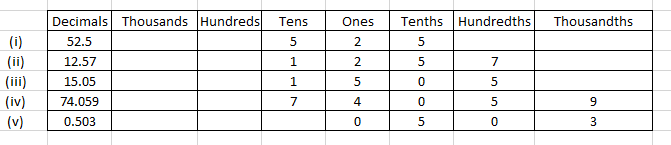2. Write the decimals shown in the following place value table: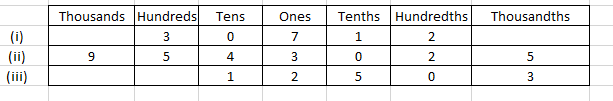Solution:
(i) We can write it as 307.12
(ii) We can write it as 9543.025
(iii) We can write it as 12.503
3. Write each of the following decimals in words:
(i) 175.04
(ii) 0.21
(iii) 9.004
(iv) 0.459
Solution:
(i) 175.04 can be written as one hundred seventy five and four hundredths.
(ii) 0.21 can be written as zero and twenty one hundredths.
(iii) 9.004 can be written as nine and four thousandths.
(iv) 0.459 can be written as zero and four hundred fifty nine thousandths.
4. Write each of the following as decimals:
(i) 65 + 2/10 + 7/100
(ii) 45 + 9/100
(iii) 88 + 5/10 + 2/1000
(iv) 3/10 + 7/1000
Solution:
(i) 65 + 2/10 + 7/100
In the above question
We know that
6 tens, 5 ones and 7 hundredths
Hence, the decimal number is 65.27.
(ii) 45 + 9/100
In the above question
We know that
4 tens, 5 ones and 9 hundredths
Hence, the decimal number is 45.09.
(iii) 88 + 5/10 + 2/1000
In the above question
We know that
8 tens, 8 ones, 5 tenths and 2 thousandths
Hence, the decimal number is 88.502.
(iv) 3/10 + 7/1000
In the above question
We know that
3 tenths, 5 ones and 7 hundredths
Hence, the decimal number is 0.307.
5. Write each of the following as decimals:
(i) Five and four tenths
(ii) Twelve and four hundredths
(iii) Nine and Seven hundred five thousandths
(iv) Zero point five two six
(v) Forty seven and six thousandths
(vi) Eight thousandths
(vii) Nineteen and nineteen hundredths.
Solution:
(i) Five and four tenths
It can be written as
5 + 4/10 = 5.4
(ii) Twelve and four hundredths
It can be written as
12 + 4/100 = 12.04
(iii) Nine and Seven hundred five thousandths
It can be written as
9 + 705/1000 = 9.705
(iv) Zero point five two six
It can be written as
0.526
(v) Forty seven and six thousandths
It can be written as
47 + 6/1000 = 47.006
(vi) Eight thousandths
It can be written as
8/1000 = 0.008
(vii) Nineteen and nineteen hundredths
It can be written as
19 + 19/100 = 19.19

Exercise 7.2 page: 7.9
1. Write each of the following as decimals:
(i) Three tenths
(ii) Two ones and five tenths
(iii) Thirty and one tenths
(iv) Twenty two and six tenths
(v) One hundred, two ones and three tenths
Solution:
(i) Three tenths
It can be written as
3/10 = 0.3
(ii) Two ones and five tenths
It can be written as
2 + 5/10 = 2.5
(iii) Thirty and one tenths
It can be written as
30 + 1/10 = 30.1
(iv) Twenty two and six tenths
It can be written as
22 + 6/10 = 22.6
(v) One hundred, two ones and three tenths
It can be written as
100 + 2 + 3/10 = 102.3
2. Write each of the following as decimals:
(i) 30 + 6 + 2/10
(ii) 700 + 5 + 7/10
(iii) 200 + 60 + 5 + 1/10
(iv) 200 + 70 + 9 + 5/10
Solution:
(i) 30 + 6 + 2/10
In the above question
We know that
3 tens, 6 ones and 2 tenths
Hence, the decimal is 36.2.
(ii) 700 + 5 + 7/10
In the above question
We know that
7 hundreds, 5 ones and 7 tenths
Hence, the decimal is 705.7.
(iii) 200 + 60 + 5 + 1/10
In the above question
We know that
2 hundreds, 6 tens, 5 ones and 1 tenths.
Hence, the decimal is 265.1.
(iv) 200 + 70 + 9 + 5/10
In the above question
We know that
2 hundreds, 7 tens, 9 ones and 5 tenths
Hence, the decimal is 279.5.
3. Write each of the following as decimals:
(i) 22/10
(ii) 3/2
(iii) 2/5
Solution:
(i) 22/10
Here the denominator is ten
Hence, the decimal is 2.2
(ii) 3/2
Multiplying the fraction by 5
We get
(3/2) × (5/5) = 15/10 = 1.5
(iii) 2/5
Multiplying the fraction by 10
We get
(2/5) × (2/2) = 4/10 = 0.4
4. Write each of the following as decimals:
(i) 40 2/5
(ii) 39 2/10
(iii) 4 3/5
(iv) 25 1/2
Solution:
(i) 40 2/5
In order to write in decimal we should make the denominator 10
So we get
40 + [(2/5) × (2/2)] = 40 + 4/10 = 40.4
(ii) 39 2/10
It can be written as
39 + 2/10 = 39 + 0.2 = 39.2
(iii) 4 3/5
In order to write in decimal we should make the denominator 10
So we get
4 + [(3/5) × (2/2)] = 4 + 6/10 = 4.6
(iv) 25 1/2
In order to write in decimal we should make the denominator 10
So we get
25+ [(1/2) × (5/5)] = 25 + 5/10 = 25.5
5. Write the following decimals as fractions. Reduce the fractions to lowest form:
(i) 3.8
(ii) 21.2
(iii) 6.4
(iv) 1.0
Solution:
(i) 3.8
It can be written as
= 3 + 8 tenths
On further calculation
= 3 + 8/10
We get
= 3(10/10) + 8/10
By further simplification
= 30/10 + 8/10
= 38/10
So we get
= 19/5
(ii) 21.2
It can be written as
= 21 + 2 tenths
On further calculation
= 21 + 2/10
We get
= 21(10/10) + 2/10
By further simplification
= 210/10 + 2/10
= 212/10
So we get
= 106/5
(iii) 6.4
It can be written as
= 6 + 4 tenths
On further calculation
= 6 + 4/10
We get
= 6(10/10) + 4/10
By further simplification
= 60/10 + 4/10
= 64/10
So we get
= 32/5
(iv) 1.0
Here, the number after decimal is zero so the fraction is 1.
6. Represent the following decimal numbers on the number line:
(i) 0.2
(ii) 1.9
(iii) 1.1
(iv) 2.5
Solution:
(i) 0.2 can be represented on the number line as given below: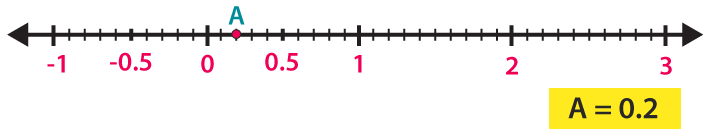(ii) 1.9 can be represented on the number line as given below: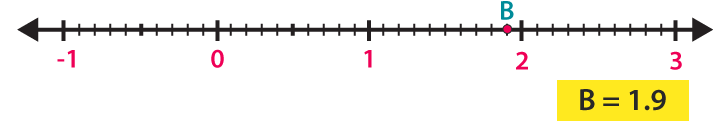(iii) 1.1 can be represented on the number line as given below: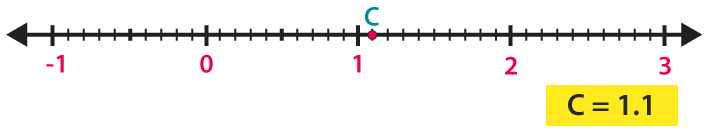(iv) 2.5 can be represented on the number line as given below: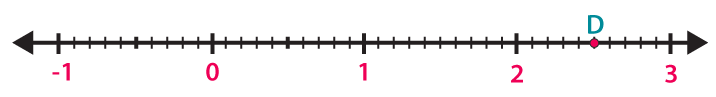7. Between which two whole numbers on the number line are the given numbers? Which one is nearer the number?
(i) 0.8
(ii) 5.1
(iii) 2.6
(iv) 6.4
(v) 9.0
(vi) 4.9
Solution:
(i) We know that
0.8 is 8 units from 0 and 2 units from 1
Hence, it is nearer to 1.
(ii) We know that
5.1 is 1 unit from 5 and 9 units from 6
Hence, it is nearer to 5.
(iii) We know that
2.6 is 6 units from 2 and 4 units from 3
Hence, it is nearer to 3.
(iv) We know that
6.4 is 4 units from 6 and 6 units from 7
Hence, it is nearer to 6.
(v) We know that
9.0 is a whole number
Hence, it is nearer to 9.
(vi) We know that
4.9 is 9 units from 4 and 1 unit from 5
Hence, it is nearer to 5.
8. Write the decimal number represented by the points on the given number line: A, B, C, D.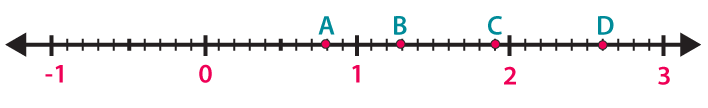Solution:
A – We know that A is at eighth place between the numbers 0 and 1
Hence, the decimal is 0.8
B – We know that B is at third place between the numbers 1 and 2
Hence, the decimal is 1.3
C – We know that C is at second place between the numbers 2 and 3
Hence, the decimal is 2.2
D – We know that D is at ninth place between the numbers 2 and 3

Hence, the decimal is 2.9
Exercise 7.3 page: 7.14
1. Write each of the following as decimals:
(i) Five hundred twenty five and forty hundredths.
(ii) Twelve and thirty five thousandths
(iii) Fifteen and seventeen thousandths
(iv) Eighty eight and forty eight hundredths
Solution:
(i) Five hundred twenty five and forty hundredths
It can be written as
525 + 40/100 = 525.40
(ii) Twelve and thirty five thousandths
It can be written as
12 + 35/1000 = 12.035
(iii) Fifteen and seventeen thousandths
It can be written as
15 + 17/1000 = 15.017
(iv) Eighty eight and forty eight hundredths
It can be written as
88 + 48/100 = 88.48
2. Write each of the following as decimals:
(i) 137 + 5/100
(ii) 20 + 9 + 4/100
Solution:
(i) 137 + 5/100
We know that
1 hundred, 3 tens, 7 ones and 5 hundredths
Hence, the decimal is 137.05.
(ii) 20 + 9 + 4/100
We know that
2 tens, 9 ones and 4 hundredths
Hence, the decimal is 29.04.
3. Write each of the following as decimals:
(i) 8/100
(ii) 300/1000
(iii) 18/1000
(iv) 208/100
(v) 888/1000
Solution:
(i) 8/100
We know that 8/100 = 0.08
Hence, the decimal is 0.08.
(ii) 300/1000
We know that 300/1000 = 3/10
So 3 tenths
Hence, the decimal is 0.3.
(iii) 18/1000
We know that which is eighteen thousandths
18/1000 = 0.018
Hence, the decimal is 0.018.
(iv) 208/100
We know that 208/100 = 200/100 + 8/100
So 2 and 8 hundredths
Hence, the decimal is 2.08.
(v) 888/1000
We know that 888/1000 = 800/1000 + 80/1000 + 8/1000
We get
= 8/10 + 8/100 + 8/1000
So 8 tenths, 8 hundredths and 8 thousands
Hence, the decimal is 0.888.
4. Write each of the following as decimals:
(i) 12 1/4
(ii) 7 1/8
(iii) 5 1/20
Solution:
(i) 12 1/4
It can be written as
= 12 + 1/4
In order to make denominator 100
Multiply and divide by 25
We get
= 12 + [(1/4) × (25/25)]
On further calculation
= 12 + 25/100
So we get
= 12.25
(ii) 7 1/8
It can be written as
= 7 + 1/8
In order to make denominator 1000
Multiply and divide by 125
We get
= 7 + [(1/8) × (125/125)]
On further calculation
= 7 + 125/1000
So we get
= 7.125
(iii) 5 1/20
It can be written as
= 5 + 1/20
In order to make denominator 100
Multiply and divide by 5
We get
= 5 + [(1/20) × (5/5)]
On further calculation
= 5 + 5/100
So we get
= 5.05
5. Write each of the following decimals as fractions. Reduce the fractions to lowest form:
(i) 0.04
(ii) 2.34
(iii) 0.342
(iv) 1.20
(v) 17.38
Solution:
(i) 0.04
It can be written as
= 0 + 0.04
We get
= 0 + 4 hundredths
On further calculation
= 0 + 4/100
So we get
= 1/25
(ii) 2.34
It can be written as
= 2 + 0.34
We get
= 2 + 34 hundredths
On further calculation
= 2 + 34/100
So we get
= 2 × (100/100) + 34/100
By further simplification
= 200/100 + 34/100
= 234/100
By division
= 117/50
(iii) 0.342
It can be written as
= 0 + 0.342
We get
= 0 + 342 thousandths
On further calculation
= 342/1000
So we get
= 171/500
(iv) 1.20
It can be written as
= 1 + 0.20
We get
= 1 + 20 hundredths
On further calculation
= 100/100 + 20/100
So we get
= 120/100
By division
= 6/5
(v) 17.38
It can be written as
= 17 + 0.38
We get
= 17 + 38 hundredths
On further calculation
= 17 + 38/100
So we get
= 17 × (100/100) + 38/100
By further simplification
= 1700/100 + 38/100
= 1738/100
By division
= 869/50
6. Write each of the following as decimals:
(i) 20 + 9 + 40/10 + 1/100
(ii) 30 + 4/10 + 8/100 + 3/1000
(iii) 137 + 5/100
(iv) 7/10 + 6/100 + 4/1000
(v) 23 + 2/10 + 6/1000
(vi) 700 + 20 + 5 + 9/100
Solution:
(i) 20 + 9 + 40/10 + 1/100
We know that
2 tens, 9 ones, 4 tenths and 1 hundredths
Hence, the decimal is 29.41.
(ii) 30 + 4/10 + 8/100 + 3/1000
We know that
3 tens, 4 tenths, 8 hundredths and 3 thousandths
Hence, the decimal is 30.483.
(iii) 137 + 5/100
We know that
1 hundred, 3 tens, 7 ones and 5 hundredths
Hence, the decimal is 137.05.
(iv) 7/10 + 6/100 + 4/1000
We know that
7 tenths, 6 hundredths and 4 thousandths
Hence, the decimal is 0.764.
(v) 23 + 2/10 + 6/1000
We know that
2 tens, 3 ones, 2 tenths and 6 thousandths
Hence, the decimal is 23.206.
(vi) 700 + 20 + 5 + 9/100
We know that
7 hundreds, 2 tens, 5 ones and 9 hundredths
Hence, the decimal is 725.09.

Exercise 7.4 page: 7.4
1. Express the following fractions as decimals:
(i) 23/10
(ii) 139/100
(iii) 4375/1000
(iv) 12 1/2
(v) 75 1/4
(vi) 25 1/8
(vii) 18 3/24
(viii) 39 7/35
(ix) 15 1/25
(x) 111/250
Solution:
(i) 23/10
It can be written as
= 20 + 3/10
We get
= 20/10 + 3/10
= 2 + 3/10
So we get
= 2.3
(ii) 139/100
It can be written as
= 100 + 30 + 9/100
We get
= 100/100 + 30/100 + 9/100
= 1 + 3/10 + 9/100
So we get
= 1.39
(iii) 4375/1000
It can be written as
= 4000 + 300 + 70 + 5/1000
We get
= 4000/1000 + 300/1000 + 70/1000 + 5/1000
= 4 + 3/10 + 7/100 + 5/1000
So we get
= 4.375
(iv) 12 1/2
It can be written as
= 12 + 1/2
Multiplying and dividing by 5 to get denominator as 10
= 12 + [(1/2) × (5/5)]
On further calculation
= 12 + 5/10
So we get
= 12.5
(v) 75 1/4
It can be written as
= 75 + 1/4
Multiplying and dividing by 25 to get 100 as denominator
= 75 + [(1/4) × (25/25)]
On further calculation
= 75 + 25/100
= 75.25
(vi) 25 1/8
It can be written as
= 25 + 1/8
Multiplying and dividing by 125 to get 1000 as denominator
= 25 + [(1/8) × (125/125)]
On further calculation
= 25 + 125/1000
= 25.125
(vii) 18 3/24
It can be written as
= 18 + 3/24
We get
= 18 + 1/8
Multiplying and dividing by 125 to get 1000 as denominator
= 18 + [(1/8) × (125/125)]
On further calculation
= 18 + 125/1000
= 18.125
(viii) 39 7/35
It can be written as
= 39 + 7/35
We get
= 39 + 1/5
Multiplying and dividing by 2 to get 10 as denominator
= 39 + [(1/5) × (2/2)]
On further calculation
= 39 + 2/10
= 39.2
(ix) 15 1/25
It can be written as
= 15 + 1/25
Multiplying and dividing by 4 to get 100 as denominator
= 15 + [(1/25) × (4/4)]
On further calculation
= 15 + 4/100
= 15.04
(x) 111/250
It can be written as
= 111 × [(1/250) × (4/4)]
On further calculation
= 444/1000
By division
= 0.444
2. Express the following decimals as fractions in the lowest form:
(i) 0.5
(ii) 2.5
(iii) 0.60
(iv) 0.18
(v) 5.25
(vi) 7.125
(vii) 15.004
(viii) 20.375
(ix) 600.75
(x) 59.48
Solution:
(i) 0.5
It can be written as
= 5/10
By division
= 1/2
(ii) 2.5
It can be written as
= 25/10
By division
= 5/2
(iii) 0.60
It can be written as
= 60/100
By division
= 3/5
(iv) 0.18
It can be written as
= 18/100
By division
= 9/50
(v) 5.25
It can be written as
= 525/100
By division
= 21/4
(vi) 7.125
It can be written as
= 7125/1000
By division
= 201/8
(vii) 15.004
It can be written as
= 15004/1000
By division
= 3751/250
(viii) 20.375
It can be written as
= 20375/1000
By division
= 163/8
(ix) 600.75
It can be written as
= 60075/100
By division
= 2403/4
(x) 59.48
It can be written as
= 5948/100
By division
= 1487/25

Exercise 7.5 page: 7.20
1. Fill in the blanks by using > or < to complete the following:
(i) 25.35 …… 8.47
(ii) 20.695 …… 20.93
(iii) 0.39 ……. 0.72
(iv) 0.109 ……. 0.83
(v) 0.236 ……. 0.201
(vi) 0.93 ……. 0.99
Solution:
(i) 25.35 …… 8.47
We know that the whole numbers 25 > 8
Hence, 25.35 > 8.47.
(ii) 20.695 …… 20.93
We know that both the whole numbers are equal
So by checking the tenth parts we know that 9 > 6
Hence, 20.695 < 20.93.
(iii) 0.39 ……. 0.72
We know that both the whole numbers are 0
So by checking the tenth parts we know that 7 > 2
Hence, 0.39 < 0.72.
(iv) 0.109 ……. 0.83
We know that both the whole numbers are 0
So by checking the tenth parts we know that 1 < 8
Hence, 0.109 < 0.83.
(v) 0.236 ……. 0.201
We know that both the whole numbers are 0
So by checking the hundredth parts we know that 3 > 0
Hence, 0.236 > 0.201.
(vi) 0.93 ……. 0.99
We know that both the whole numbers are 0
So by checking the hundredth parts we know that 3 < 9
Hence, 0.93 < 0.99.
(i) 1.008 or 1.800
(ii) 3.3 or 3.300
(iii) 5.64 or 5.603
(iv) 1.5 or 1.50
(v) 1.431 or 1.439
(vi) 0.5 or 0.05
Solution:
(i) 1.008 or 1.800
We know that the whole numbers are equal
So by comparing tenth parts we know that 0 < 8
Hence, 1.008 < 1.800.
(ii) 3.3 or 3.300
We know that the whole numbers and tenths place are equal
Hence, 3.3 = 3.300.
(iii) 5.64 or 5.603
We know that the whole numbers are equal
So by comparing the hundredth place we know that 4 > 0
Hence, 5.64 > 5.603.
(iv) 1.5 or 1.50
We know that the whole numbers and tenths place are equal
Hence, 1.5 = 1.50.
(v) 1.431 or 1.439
We know that the whole numbers are equal
So by comparing thousandths place we know that 1 < 9
Hence, 1.431 < 1.439.
(vi) 0.5 or 0.05
We know that the whole numbers are equal
So by comparing tenth place we know that 5 > 0
Hence, 0.5 > 0.05.

Exercise 7.6 page: 7.24
1. Express as Rupees (Rs) using decimals:
(i) 15 paisa
(ii) 5 paisa
(iii) 350 paisa
(iv) 2 rupees 60 paisa
Solution:
(i) 15 paisa
We know that 100 paisa = Rs 1
So we get 1 paisa = Rs 1/100
It can be written as
15 paisa = 15/100
We get
15 paisa = Rs 0.15
(ii) 5 paisa
We know that 100 paisa = Rs 1
So we get 1 paisa = Rs 1/100
It can be written as
5 paisa = 5/100
We get
5 paisa = Rs 0.05
(iii) 350 paisa
We know that 100 paisa = Rs 1
So we get 1 paisa = Rs 1/100
It can be written as
350 paisa = 350/100
We get
350 paisa = Rs 3.50
(iv) 2 rupees 60 paisa
We know that 100 paisa = Rs 1
So we get 1 paisa = Rs 1/100
It can be written as
2 rupees 60 paisa = 2 + 60/100
We get
2 rupees 60 paisa = Rs 2.60
2. Express as metres (m) using decimals:
(i) 15 cm
(ii) 8 cm
(iii) 135 cm
(iv) 3 m 65 cm
Solution:
(i) 15 cm
We know that 100 cm = 1 m
So we get 1 cm = 1/100 m
It can be written as
15 cm = 15 (1/100)
We get
15 cm = 0.15 m
(ii) 8 cm
We know that 100 cm = 1 m
So we get 1 cm = 1/100 m
It can be written as
8 cm = 8 (1/100)
We get
8 cm = 0.08 m
(iii) 135 cm
We know that 100 cm = 1 m
So we get 1 cm = 1/100 m
It can be written as
135 cm = 135 (1/100)
We get
135 cm = 1.35 m
(iv) 3 m 65 cm
We know that 100 cm = 1 m
So we get 1 cm = 1/100 m
It can be written as
3 m 65 cm = 3 + 65 (1/100)
We get
3 m 65 cm = 3.65 m
3. Express as centimeter (cm) using decimals:
(i) 5 mm
(ii) 60 mm
(iii) 175 mm
(iv) 4 cm 5 mm
Solution:
(i) 5 mm
We know that 10 mm = 1 cm
So we get 1 mm = 1/10 cm
It can be written as
5 mm = 5/10
We get
5 mm = 0.5 cm
(ii) 60 mm
We know that 10 mm = 1 cm
So we get 1 mm = 1/10 cm
It can be written as
60 mm = 60/10
We get
60 mm = 6 cm
(iii) 175 mm
We know that 10 mm = 1 cm
So we get 1 mm = 1/10 cm
It can be written as
175 mm = 175/10
We get
175 mm = 17.5 cm
(iv) 4 cm 5 mm
We know that 10 mm = 1 cm
So we get 1 mm = 1/10 cm
It can be written as
4 cm 5 mm = 4 + 5/10
We get
4 cm 5 mm = 4.5 cm
4. Express as kilometer (km) using decimals:
(i) 5 m
(ii) 55 m
(iii) 555 m
(iv) 5555 m
(v) 15 km 35 m
Solution:
(i) 5 m
We know that 1000 m = 1 km
So we get 1 m = 1/1000 km
It can be written as
5 m = 5/1000 km
We get
5 m = 0.005 km
(ii) 55 m
We know that 1000 m = 1 km
So we get 1 m = 1/1000 km
It can be written as
55 m = 55/1000 km
We get
55 m = 0.055 km
(iii) 555 m
We know that 1000 m = 1 km
So we get 1 m = 1/1000 km
It can be written as
555 m = 555/1000 km
We get
555 m = 0.555 km
(iv) 5555 m
We know that 1000 m = 1 km
So we get 1 m = 1/1000 km
It can be written as
5555 m = 5555/1000 km
We get
5555 m = 5.555 km
(v) 15 km 35 m
We know that 1000 m = 1 km
So we get 1 m = 1/1000 km
It can be written as
15 km 35 m = 15 + 35/1000 km
We get
15 km 35 m = 15.035 km
5. Express as kilogram (kg) using decimals:
(i) 8 g
(ii) 150 g
(iii) 2750 g
(iv) 5 kg 750 g
(v) 36 kg 50 g
Solution:
(i) 8 g
We know that 1000 g = 1 kg
So we get 1 g = 1/1000 kg
It can be written as
8 g = 8/1000
We get
8 g = 0.008 kg
(ii) 150 g
We know that 1000 g = 1 kg
So we get 1 g = 1/1000 kg
It can be written as
150 g = 150/1000
We get
150 g = 0.150 kg
(iii) 2750 g
We know that 1000 g = 1 kg
So we get 1 g = 1/1000 kg
It can be written as
2750 g = 2750/1000
We get
2750 g = 2.750 kg
(iv) 5 kg 750 g
We know that 1000 g = 1 kg
So we get 1 g = 1/1000 kg
It can be written as
5 kg 750 g = 5 + 750/1000
We get
5 kg 750 g = 5.750 kg
(v) 36 kg 50 g
We know that 1000 g = 1 kg
So we get 1 g = 1/1000 kg
It can be written as
36 kg 50 g = 36 + 50/1000
We get
36 kg 50 g = 36.050 kg
6. Express each of the following without using decimals:
(i) Rs 5.25
(ii) 8.354 kg
(iii) 3.5 cm
(iv) 3.05 km
(v) 7.54 m
(vi) 15.005 kg
(vii) 12.05 m
(viii) 0.2 cm
Solution:
(i) Rs 5.25
We know that 100 paisa = 1 rupee
So we get 1 paisa = 1/100 rupee
It can be written as
Rs 5.25 = 5 + 25/100
We get
Rs 5.25 = 5 + 1/4
On further calculation
Rs 5.25 = Rs 21/4
(ii) 8.354 kg
We know that 100 g = 1 kg
So we get 1 g = 1/1000 kg
It can be written as
8.354 kg = 8354/1000 kg
(iii) 3.5 cm
We know that 10 mm = 1 cm
So we get 1 mm = 1/10 cm
It can be written as
3.5 cm = 3 + 5/10
On further calculation
3.5 cm = 3 + 1/2
We get
3.5 cm = 7/2 cm
(iv) 3.05 km
We know that 1000 m = 1 km
So we get 1 m = 1/1000 km
It can be written as
3.05 km = 3 + 5/100
Multiplying and dividing by 10
3.05 km = 3 + 50/1000
On further calculation
3.05 km = 3 + 1/20
We get
3.05 km = 61/20 km
(v) 7.54 m
We know that 1000 m = 1 km
So we get 1 m = 1/1000 km
It can be written as
7.54 m = 7 + 54/100
On further calculation
7.54 m = 7 + 27/50
We get
7.54 m = 377/50 m
(vi) 15.005 kg
We know that 1 kg = 10000 g
So we get 1 g = 1/1000 kg
It can be written as
15.005 kg = 15 + 5/1000
On further calculation
15.005 kg = 15 + 1/200
We get
15.005 kg = 3001/200 kg
(vii) 12.05 m
We know that 1 m = 100 cm
So we get 1 cm = 1/100 m
It can be written as
12.05 m = 12 + 5/100
On further calculation
12.05 m = 12 + 1/20
We get
12.05 m = 241/20 m
(viii) 0.2 cm
We know that 10 mm = 1 cm
So we get 1 mm = 1/10 cm
It can be written as
0.2 cm = 0 + 2/10
On further calculation
0.2 cm = 1/5 cm

Exercise 7.7 page: 7.26
1. Choose the decimal (s) from the brackets which is (are) not equivalent to the given decimals:
(i) 0.8 (0.80, 0.85, 0.800, 0.08)
(ii) 25.1 (25.01, 25.10, 25.100, 25.001)
(iii) 45.05 (45.050, 45.005, 45.500, 45.0500)
Solution:
(i) 0.8 (0.80, 0.85, 0.800, 0.08)
We know that 0.85 and 0.08 are not equivalent to the given decimal
For 0.85, 5 is in the hundredth place and for 0.8 the value is 0.
For 0.08, 0 is in the tenth place and for 0.8 the value is 8.
(ii) 25.1 (25.01, 25.10, 25.100, 25.001)
For 25.01, 0 is in the tenth place and for 25.001 the value is 0.
(iii) 45.05 (45.050, 45.005, 45.500, 45.0500)
For 45.005, 0 is in the hundredth place and for 45.05 the value is 5
For 45.500, 5 is in the tenth place and for 45.05 the value is 0.
2. Which of the following are like decimals:
(i) 0.34, 0.07, 5.35, 24.70
(ii) 45.05, 4.505, 20.55, 20.5
(iii) 8.80, 17.08, 8.94, 0.27
(iv) 4.50, 16.80, 0.700, 7.08
Solution:
(i) 0.34, 0.07, 5.35, 24.70
The given values are like decimals because equal number of digits are present after the decimal point.
(ii) 45.05, 4.505, 20.55, 20.5
The given values are unlike decimals because different number of digits are present after the decimal point.
(iii) 8.80, 17.08, 8.94, 0.27
The given values are like decimals because equal number of digits are present after the decimal point.
(iv) 4.50, 16.80, 0.700, 7.08
The given values are unlike decimals because different number of digits are present after the decimal point.
3. Which of the following statements are correct?
(i) 8.05 and 7.95 are like decimals.
(ii) 0.95, 0.306, 7.10 are unlike decimals.
(iii) 3.70 and 3.7 are like decimals.
(iv) 13.59, 1.359, 135.9 are like decimals.
(v) 5.60, 3.04, 0.45 are like decimals.
Solution:
(i) Correct because the two decimals have same number of digits present after decimal point.
(ii) Correct because the three decimals have same number of digits present after decimal point.
(iii) Incorrect because the two decimals have different number of digits present after decimal point.
(iv) Incorrect because the three decimals have different number of digits present after decimal point.
(v) Correct because the three decimals have same number of digits present after decimal point.
4. Convert each of the following sets of unlike decimals to like decimal:
(i) 7.8, 7.85
(ii) 2.02, 3.2
(iii) 0.6, 5.8, 12.765
(iv) 5.296, 5.2, 5.29
(v) 4.3294, 43.29, 432.94
Solution:
(i) 7.8, 7.85
In the given values we know that 7.85 contains 2 digits after decimal point so by changing 7.8 as 7.80
Hence, 7.80 and 7.85 are like decimals.
(ii) 2.02, 3.2
In the given values we know that 2.02 contains 2 digits after decimal point so by changing 3.2 as 3.20
Hence, 2.02 and 3.20 are like decimals.
(iii) 0.6, 5.8, 12.765
In the given values we know that 12.765 contains 3 digits after decimal point so by changing 0.6 as 0.600 and 5.8 as 5.800
Hence, 0.600, 5.800 and 12.765 are like decimals.
(iv) 5.296, 5.2, 5.29
In the given values we know that 5.296 contains 3 digits after decimal point so by changing 5.2 as 5.200 and 5.29 as 5.290
Hence, 5.296, 5.200 and 5.290 are like decimals.
(v) 4.3294, 43.29, 432.94
In the given values we know that 4.3294 contains 4 digits after decimal point so by changing 43.29 as 43.2900 and 432.94 as 432.9400
Hence, 4.3294, 43.2900 and 432.9400 are like decimals.

Exercise 7.8 page: 7.28
1. Find the sum in each of the following: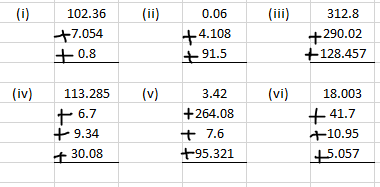Solution:
(i) We know that
102.360 + 7.054 + 0.800 = 110.214
(ii) We know that
0.060 + 4.108 + 91.500 = 95.668
(iii) We know that
312.800 + 290.020 + 128.457 = 731.277
(iv) We know that
113.285 + 6.700 + 9.340 + 30.080 = 370.421
(v) We know that
18.003 + 41.700 + 10.950 + 5.057 = 75.710
(i) 41.8, 39.24, 5.01 and 62.6
(ii) 4.702, 4.2, 6.02 and 1.27
(iii) 18.03, 146.3, 0.829 and 5.324
Solution:
(i) 41.8, 39.24, 5.01 and 62.6
It can be written as
41.80 + 39.24 + 5.1 + 62.60 = 148.65
(ii) 4.702, 4.2, 6.02 and 1.27
It can be written as
4.702 + 4.200 + 6.020 + 1.270 = 16.192
(iii) 18.03, 146.3, 0.829 and 5.324
It can be written as
18.030 + 146.300 + 0.829 + 5.324 = 170.483
3. Find the sum in each of the following:
(i) 0.007 + 8.5 + 30.08
(ii) 280.69 + 25.2 + 38
(iii) 25.65 + 9.005 + 3.7
(iv) 27.076 + 0.55 + 0.004
Solution:
(i) 0.007 + 8.5 + 30.08
It can be written as
0.007 + 8.500 + 30.080 = 38.587
(ii) 280.69 + 25.2 + 38
It can be written as
280.69 + 25.20 + 38.00 = 343.89
(iii) 25.65 + 9.005 + 3.7
It can be written as
25.650 + 9.005 + 3.700 = 38.355
(iv) 27.076 + 0.55 + 0.004
It can be written as
27.076 + 0.550 + 0.004 = 27.630
4. Radhika’s mother gave her Rs 10.50 and her father gave her Rs 15.80, find the total amount given to Radhika by her parents.
Solution:
Amount given by Radhika’s mother = Rs 10.50
Amount given by Radhika’s father = Rs 15.80
So the total amount given by her parents = Rs 10.50 + Rs 15.80 = Rs 26.30
Hence, the total amount given by her parents is Rs 26.30.
5. Rahul bought 4 kg 90 g apples, 2 kg 60 g of grapes and 5 kg 300 g of mangoes. Find the weight of the fruits he bought in all.
Solution:
Weight of apples bought by Rahul = 4 kg 90 g = 4.090 kg
Weight of grapes bought by Rahul = 2 kg 60 g = 2.060 kg
Weight of mangoes bought by Rahul = 5 kg 300 g = 5.300 kg
So the weight of all the fruits = 4.090 + 2.060 + 5.300 = 11.450 kg
Hence, the weight of the fruits bought by Rahul is 11.450 kg.
6. Nasreen bought 3 m 20 cm cloth for her shirt and 2 m 5 cm cloth for skirt. Find the total cloth bought by her.
Solution:
Cloth bought by Nasreen for shirt = 3 m 20 cm = 3.20 m
Cloth bought by Nasreen for skirt = 2 m 50 cm = 2.05 m
So the total cloth bought by her = 3.20 + 2.05 = 5.25 m = 5 m 25 cm
Hence, the total cloth bought by her is 5 m 25 cm.
7. Sunita travels 15 km 268 m by bus, 7 km 7 m by car and 500 m by foot in order to reach her school. How far is her school from her residence?
Solution:
Distance travelled by Sunita by bus = 15 km 268 m = 15.268 km
Distance travelled by Sunita by car = 7 km 7 m = 7.007 km
Distance travelled by Sunita by foot = 500 m = 0.500 km
So the distance from residence to school = 15.268 + 7.007 + 0.500 = 22.775 km
Hence, the distance from her residence to school is 22.775 km.

Exercise 7.9 page: 7.31
1. Subtract: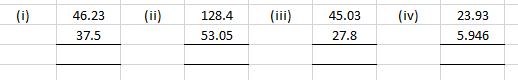Solution:
(i) We know that
46.23 – 37.5 = 8.73
(ii) We know that
128.4 – 53.05 = 75.35
(iii) We know that
45.03 – 27.8 = 17.23
(iv) We know that
23.93 – 5.946 = 17.984
2. Find the value of:
(i) 9.756 – 6.28
(ii) 21.05 – 15.27
(iii) 18.5 – 6.79
(iv) 48.1 – 0.37
(v) 108.032 – 86.8
(vi) 91.001 – 72.9
(vii) 32.7 – 25.86
(viii) 100 – 26.32
Solution:
(i) 9.756 – 6.28
We know that
9.756 – 6.280 = 3.476
(ii) 21.05 – 15.27
We know that
21.05 – 15.27 = 5.78
(iii) 18.5 – 6.79
We know that
18.50 – 6.79 = 11.71
(iv) 48.1 – 0.37
We know that
48.10 – 0.37 = 47.73
(v) 108.032 – 86.8
We know that
108.032 – 86.800 = 21.232
(vi) 91.001 – 72.9
We know that
91.001 – 72.900 = 18.101
(vii) 32.7 – 25.86
We know that
32.70 – 25.86 = 6.84
(viii) 100 – 26.32
We know that
100 – 26.32 = 73.68
3. The sum of two numbers is 100. If one of them is 78.01, find the other.
Solution:
One of the number = 78.01
Sum of two numbers = 100
Consider x as the other number
It can be written as
78.01 + x = 100
On further calculation
x = 100 – 78.01
By subtraction
x = 21.99
Hence, the other number is 21.99.
4. Waheeda’s school is at a distance of 5 km 350 m from her house. She travels 1 km 70 m on foot and the rest she travels by bus. How much distance does she travel by bus?
Solution:
Distance of school from house = 5 km 350 m = 5.350 km
Distance travelled on foot = 1 km 70 m = 1.070 km
Consider x km as the distance travelled by bus
It can be written as
1.070 + x = 5.350
On further calculation
x = 5.350 – 1.070
So we get
x = 4.280 km
Hence, the distance travelled by bus is 4.280 km.
5. Raju bought a book for Rs 35.65. He gave Rs 50 to the shopkeeper. How much money did he get back from the shopkeeper?
Solution:
Cost of book = Rs 35.65
Amount given = Rs 50
So the balance returned = 50 – 35.65 = Rs 14.35
Hence, the balance returned by the shopkeeper is Rs 14.35.
6. Ruby bought a watermelon weighing 5 kg 200 g. Out of this she gave 2 kg 750 g to her neighbor. What is the weight of the watermelon left with Ruby?
Solution:
Weight of watermelon bought by Ruby = 5 kg 200 g = 5.200 kg
Weight of watermelon Ruby gave to neighbor = 2 kg 750 g = 2.750 kg
So the weight of watermelon left with Ruby = Weight of watermelon bought by Ruby – Weight of watermelon Ruby gave to neighbor
We get
Weight of watermelon left with Ruby = 5.200 – 2.750 = 2.450 kg
Hence, the weight of watermelon left with Ruby is 2.450 kg.
7. Victor drove 89.050 km on Saturday and 73.9 km on Sunday. How many kilometres more did he drive on Sunday?
Solution:
Distance travelled by Victor on Saturday = 89.050 km
Distance travelled by Victor on Sunday = 73.9 km
So the distance travelled more by Victor on Saturday = 89.050 – 73.9 = 15.15 km
Hence, Victor drove 15.15 km more on Saturday.
8. Raju bought a book for Rs 35.65. He gave Rs 50 to the shopkeeper. How much money did he get back from the shopkeeper?
Solution:
Cost of the book = Rs 35.65
Amount given = Rs 50
So the balance retuned = 50 – 35.65 = Rs 14.35
Hence, the shopkeeper returned back Rs 14.35.
9. Gopal travelled 125.5 km by bus, 14.25 km by pony and the rest of distance to Kedarnath on foot. If he covered a total distance of 15 km, how much did he travel on foot?
Solution:
Distance travelled by Gopal by bus = 125.5 km
Distance travelled by Gopal by pony = 14.25km
Consider x km as the distance travelled on foot
We know that
Total distance = Distance travelled by Gopal by bus + Distance travelled by Gopal by pony + Distance travelled by Gopal on foot
By substituting the values
150 = 125.5 + 14.25 + x
On further calculation
x = 150 – 125.5 – 14.25
We get
x = 10.25 km
Hence, the distance travelled by Gopal on foot is 10.25 km.
10. Tina had 20 m 5 cm long cloth. She cuts 4 m 50 cm length of cloth from this for making a curtain. How much cloth is left with her?
Solution:
Length of cloth Tina had = 20 m 5 cm = 20.05 m
Length of cloth cut to make curtain = 4m 50 cm = 4.50 m
So the length of cloth left with her = Length of cloth Tina had – Length of cloth cut to make curtain
By substituting values
The length of cloth left with her = 20.05 – 4.50 = 15.55 m
Hence, the length of cloth left with Tina is 15.55 m.
11. Vineeta bought a book for Rs 18.90, a pen for Rs 8.50 and some papers for Rs 5.05. She gave fifty rupee to the shopkeeper. How much balance did she get back?
Solution:
Cost of book = Rs 18.90
Cost of pen = Rs 8.50
Cost of papers = Rs 5.05
So the total cost = 18.90 + 8.50 + 5.05 = Rs 32.45
Amount given to the shopkeeper = Rs 50
So the balance returned back = 50 – 32.45 = Rs 17.55
Hence, the shopkeeper returned back Rs 17.55.
12. Tanuj walked 8.62 km on Monday, 7.05 km on Tuesday and some distance on Wednesday. If he walked 21.01 km in three days, how much distance did he walk on Wednesday?
Solution:
Distance walked by Tanuj on Monday = 8.62 km
Distance walked by Tanuj on Tuesday = 7.05 km
Consider x km as the distance walked by Tanuj on Wednesday
It can be written as
Total distance = Distance walked by Tanuj on Monday + Distance walked by Tanuj on Tuesday + Distance walked by Tanuj on Wednesday
By substituting the values
21.01 = 8.62 + 7.05 + x
On further calculation
x = 21.01 – 8.62 – 7.05
So we get
x = 5.34 km
Hence, the distance walked by Tanuj on Wednesday is 5.34 km.
Objective Type Questions page: 7.32
Mark the correct alternative in each of the following:
1. 3/10 is equal to
(a) 3.1
(b) 1.3
(c) 0.3
(d) 0.03
Solution:
The option (c) is correct answer.
We know that 3/10 = 0.3
Here the denominator is 10, so we have to mark the decimal where 3 is in the tenth place.
2. 7/100 is equal to
(a) 7.1
(b) 7.01
(c) 0.7
(d) 0.07
Solution:
The option (d) is correct answer.
We know that 7/100 = 0.07
Here the denominator is 100, so we have to mark the decimal where 7 is in the hundredth place.
3. 4/1000 is equal to
(a) 0.004
(b) 0.04
(c) 0.4
(d) 4.001
Solution:
The option (a) is correct answer.
Here the denominator is 1000, so we have to mark the decimal where 4 is in the thousandth place.
4. The value of 37/10000 is
(a) 0.0370
(b) 0.0037
(c) 0.00037
(d) 0.000037
Solution:
The option (b) is correct answer.
Here the denominator is 10000, so we have to mark the decimal where 3 is in the thousandth place and 7 is in the ten-thousandth place.
5. The place value of 5 in 0.04532 is
(a) 5
(b) 5/100
(c) 5/1000
(d) 5/10000
Solution:
The option (c) is correct answer.
We know that 5 is in the thousandth place.

So we get 0.04532 = 4/100 + 5/1000 + 3/10000 + 2/100000
6. The value of 231/1000 is
(a) 0.231
(b) 2.31
(c) 23.1
(d) 0.0231
Solution:
The option (a) is correct answer.
It can be written as
231/1000 = (200+30+1)/1000 = 200/1000 + 30/1000 + 1/1000 = 2/10 + 3/100 + 1/1000

Here we have 2 tenths, 3 hundredths and 1 thousandth.

Hence, the value of 231/1000 is 0.231.
7. The value of 3 5/1000 is
(a) 3.5
(b) 3.05
(c) 3.005
(d) 3.0005
Solution:
The option (c) is correct answer.
It can be written as
3 5/1000 = 3 + 5/1000 = 3 + 0.005 = 3.005
8. The value of 3/25 is
(a) 1.2
(b) 0.12
(c) 0.012
(d) None of these
Solution:
The option (b) is correct answer.
It can be written as
3/25 = (3×4)/ (25×4) = 12/100 = 0.12
9. The value of 2 1/25 is
(a) 2.4
(b) 2.25
(c) 2.04
(d) 2.40
Solution:
The option (c) is correct answer.
It can be written as
2 1/25 = 2 + 1/25 = 2 + (1×4)/ (25×4) = 2 + 4/100 = 2 + 0.04 = 2.04
10. 4 7/8 is equal to
(a) 4.78
(b) 4.87
(c) 4.875
(d) None of these
Solution:
The option (c) is correct answer.
It can be written as
4 7/8 = 4 + 7/8 = 4 + (7×125)/ (8×125)
On further calculation
4 7/8 = 4 + 875/1000 = 4 + 0.875 = 4.875
11. 2+3/10+5/100 is equal to
(a) 2.305
(b) 2.3
(c) 2.35
(d) 0.235
Solution:
The option (c) is correct answer.
We know that 3/10 = 0.3 having denominator as 10, so we need to mark the decimal where 3 is in the tenth place

5/100= 0.05 having denominator as 100, so we need to mark the decimal where 5 is in the hundredth place

It can be written as,
2 + 3/10 + 5/100 = 2+ 0.3 + 0.05 = 2.35
12. 3/100+5/10000 is equal to
(a) 0.35
(b) 0.305
(c) 0.0305
(d) 0.3005
Solution:
The option (d) is correct answer.
We know that 3/100 = 0.03 having denominator 100, so we mark the decimal where 3 is in the hundredth place

5/10000 = 0.0005 having denominator 10000, so we mark the decimal where 5 is in the ten thousandth place

It can be written as,
3/100 + 5/10000 = 0.03 + 0.0005 = 0.0305
13. 1 cm is equal is
(a) 0.1 m
(b) 0.01 m
(c) 0.10 m
(d) 0.001 m
Solution:
The option (b) is correct answer.
100 cm = 1 m

So we get,
1 cm = 1/100 m = 0.01 m
14. 1 m is equal to
(a) 0.1 km
(b) 0.01 km
(c) 0.001 km
(d) 0.0001 km
Solution:
The option (c) is correct answer.
1000 m = 1 km

So we get,
1 m = 1/1000 m = 0.001 km
15. 2 kg 5 gm is equal to
(a) 2.5 kg
(b) 2.05 kg
(c) 2.005 kg
(d) 2.6 kg
Solution:
The option (c) is correct answer.
1000 g = 1 kg

So we get 1 g = 1/1000 kg = 0.001 kg

The same way 5 g = 5/1000 kg = 0.005 kg

Hence, 2 kg 5 gm =2 kg + 0.005 kg = 2.005 kg
16. 15 litres and 15 ml is equal to
(a) 15.15 litres
(b) 15.150 litres
(c) 15.0015 litres
(d) 15.015 litres
Solution:
The option (d) is correct answer.
1000 ml = 1 litre

So we get 1 ml = 1/1000 = 0.001 litre

The same way 15 ml = 15/1000 = 0.015 litre

Hence, 15 litre and 15 ml = 15 litre + 0.015 litre = 15.015 litres
17. Which of the following are like decimals?
(a) 5.5, 5.05, 5.005, 5.50
(b) 5.5, 0.55, 5.55, 5.555
(c) 5.5, 6.6, 7.7, 8.8
(d) 0.5, 0.56, 0.567, 0.5678
Solution:
The option (c) is correct answer.
5.5. 6.6, 7.7, 8.8 are like decimals having same number of decimals.
18. The value of 0.5 + 0.005 + 5.05 is
(a) 5.55
(b) 5.555
(c) 5.055
(d) 5.550
Solution:
The option (b) is correct answer.
0.5 + 0.005 + 5.05 = 5.555
19. 0.35 − 0.035 is equal to
(a) 0.3
(b) 0.349
(c) 0.315
(d) 0.353
Solution:
The option (c) is correct answer.
0.35 – 0.035 = 0.315
20. 2.5 + 3.05 − 4.005 is equal to
(a) 1.545
(b) 1.455
(c) 1.554
(d) 0.545
Solution:
The option (a) is correct answer.
2.5 + 3.05 − 4.005 = 5.55 − 4.005 = 1.545
21. Which is greater among 2.3, 2.03, 2.33, 2.05?
(a) 2.3
(b) 2.03
(c) 2.33
(d) 2.05
Solution:
The option (c) is correct answer.
We know that the whole parts of all the above numbers are equal.
So by comparing the tenth parts, two of the decimals have a tenth part 0 and two have a tenth part 3.
Now by leaving the decimals which have a tenth part 0, 2.3 and 2.33.
By comparing them, 2.33 is greater than 2.3, where, 2.3 has no hundredth part, while 2.33, the hundredth part is 3.
Courtesy : CBSE